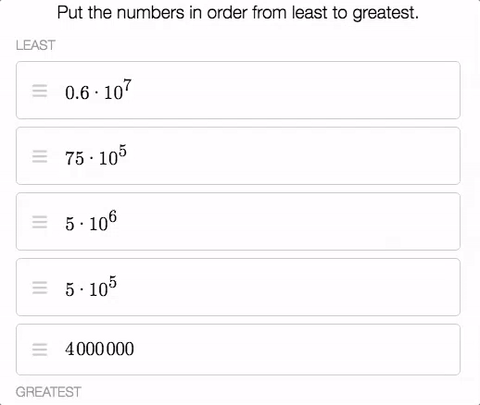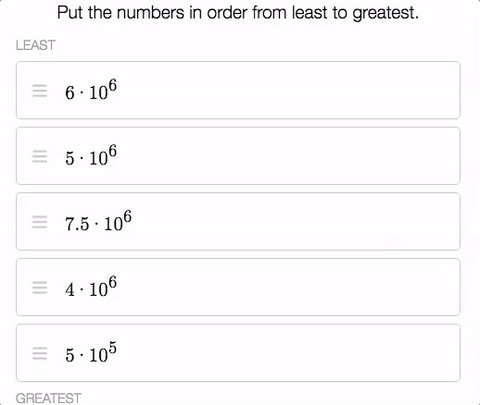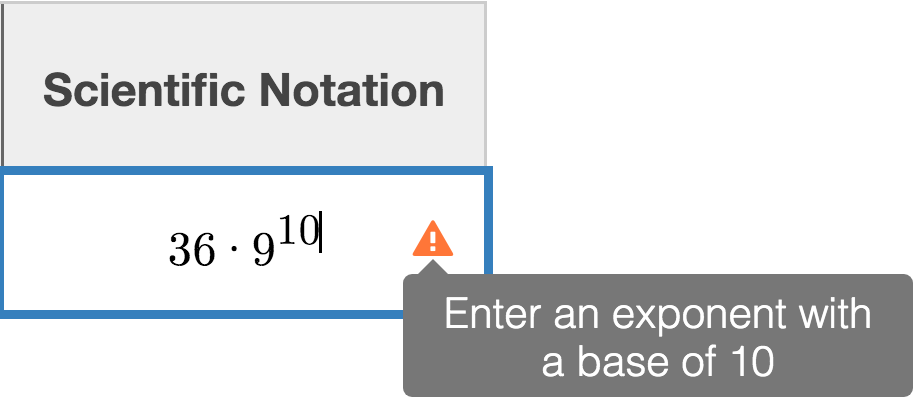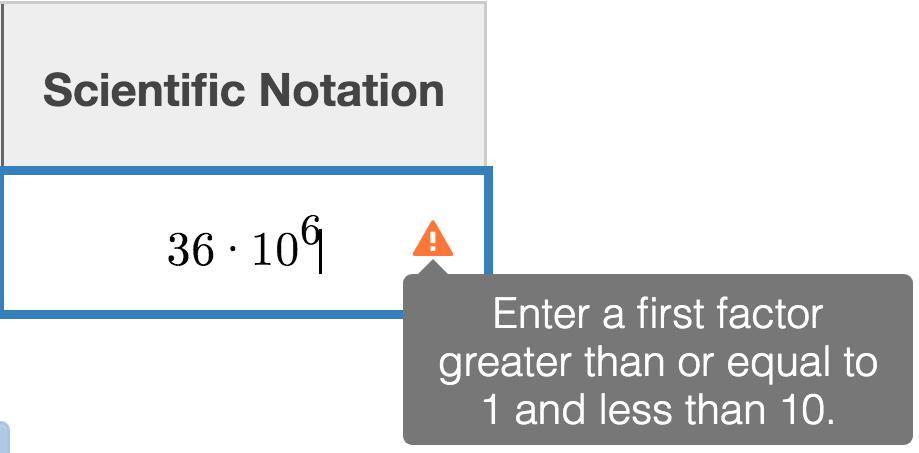Newly Released Scientific Notation Activity

03 October 2019

There are things in life that science teachers and math teachers disagree on. Is 0.1 equal to 0.10? Do all numbers require units? Is a ratio different from a rate?

But there is one thing that math and science teachers can always agree on—teaching scientific notation is a strangely difficult thing to do.

Desmos isn’t here to tell you that we’ve solved this problem, but we are here to tell you that we’ve built a thing that makes this difficult thing a bit more fun.

We have adapted some ideas from the Grade 8 Illustrative Mathematics materials (which you can download for free through Open Up Resources) to build The Solar System, Test Tubes, and Scientific Notation, which is free for you to use in your own classroom.

Scientific notation is useful for a number of things. Communicating a sense of the size of the numbers involved is one of those things, so our activity begins by asking students to sort two sets of numbers from least to greatest.We keep it a secret that the two lists are actually the same numbers until students have had a chance to sort them out.

Next up, we help students distinguish scientific notation from not-scientific notation, and then it’s time to get some practice where we offer two types of feedback. The first is about whether your numbers are in the correct form.The second type of feedback is whether the number a student has expressed in scientific notation is in fact the correct number. If it is, we’ll put their planet into orbit; if not, we’ll show them that their orbit doesn’t match their planet.

As the activity’s title suggests, we ask students to express numbers that are both large (the solar system) and small (test tubes). Plenty of practice and good, useful feedback—perhaps these are things on which math and science teachers can agree?